Question

# The Nolan Corporation finds that it is necessary to determine its marginal cost of capital. Nolan’s...

The Nolan Corporation finds that it is necessary to determine its marginal cost of capital. Nolan’s current capital structure calls for 45 percent debt, 15 percent preferred stock, and 40 percent common equity. Initially common equity will be in the form of retained earnings (Ke) and then new common stock (Kn). The costs of the various sources of financing are as follows: debt, 5.6 percent; preferred stock, 9 percent; retained earning, 12 percent; and new common stock, 13.2 percent.

a. What is the initial weighted average cost of capital? (Include debt, preferred stock, and common equity in the form of retained earnings, Ke.)

b. If the firm has \$12 million in retained earnings, at what size capital structure will the firm run out of retained earnings?

c. What will the marginal cost of capital be immediately after that point? (Equity will remain at 40 percent of the capital structure, but will all be in the form of new common stock, Kn).

d. The 5.6 percent cost of debt referred to above applies only to the first \$18 million of debt. After that the cost of debt will be 7.2 percent. At what size capital structure will there be a change in the cost of debt?

e. What will the marginal cost of capital be immediately after that point? (Consider the facts in both parts c and d.)

a.

Calculation of initial Weighted Average Cost of Capital using K e :

From the given data:

Cost of debt is 5.6%,

Cost of preferred stock is 9%,

Cost of common stock is 12%.

Weighted Average Cost of Capital is sum of all sources to finance with weight age.

Calculation of weighted Average Cost of Capita is as below: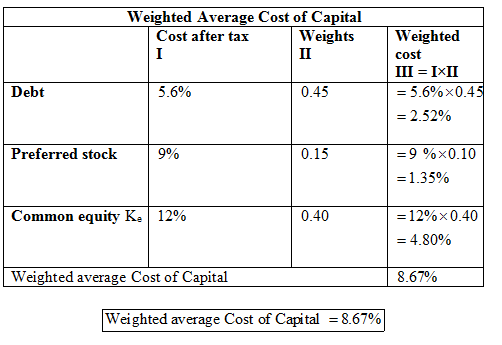b.

From the given data:

Retained earnings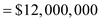Retained earnings pertain only to the common equity. As per the given: table, the Weight age of the common equity to the total capital is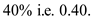The total amount of capital structure required by firm so that the firm will run out of retained earnings is: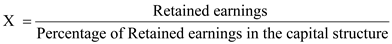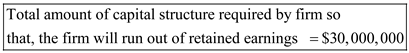c.

Calculation of marginal cost of capital using K n :

From the given data, to calculate the marginal cost of capital, the Cost of new common stock is 13.20%. remaining variables are same.

Calculation of marginal cost of capital is as below: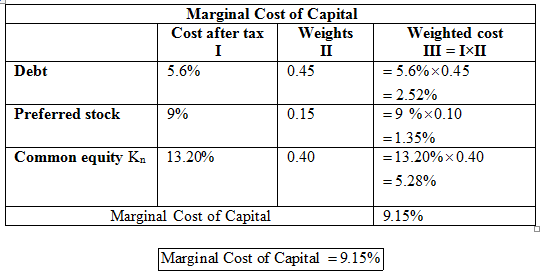d.

From the given data:

Cost of debt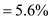This cost is applicable to the amount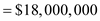Later increase in cost of debt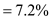As the name suggests, this refers to the debt part of the capital structure. As per the Given: table, the Weightage of the debt to the total capital is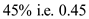. The total amount of capital structure required by firm so that there will be a change in the cost of debt is: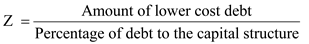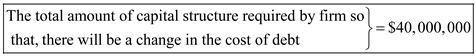e.

Calculation of marginal cost of capital using K n with new cost of debt:

From the given data:

New debt is 7.2%,

New common stock is 13.20%.

The following table indicates about the calculation of Marginal Cost of Capital with new Cost of Capital: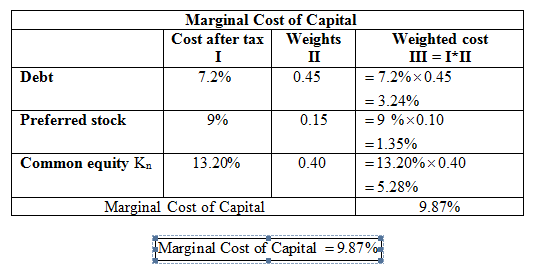#### Earn Coins

Coins can be redeemed for fabulous gifts.

Similar Homework Help Questions
• ### The Nolan Corporation finds that it is necessary to determine its marginal cost of capital. Nolan’s...

The Nolan Corporation finds that it is necessary to determine its marginal cost of capital. Nolan’s current capital structure calls for 35 percent debt, 10 percent preferred stock, and 55 percent common equity. Initially common equity will be in the form of retained earnings (Ke) and then new common stock (Kn). The costs of the various sources of financing are as follows: debt, 5.6 percent; preferred stock, 11 percent; retained earnings, 8 percent; and new common stock, 9.2 percent. a....

• ### The Nolan Corporation finds it is necessary to determine its marginal cost of capital. Nolan’s current...

The Nolan Corporation finds it is necessary to determine its marginal cost of capital. Nolan’s current capital structure calls for 50 percent debt, 30 percent preferred stock, and 20 percent common equity. Initially, common equity will be in the form of retained earnings (Ke) and then new common stock (Kn). The costs of the various sources of financing are as follows: debt, 9.6 percent; preferred stock, 9 percent; retained earnings, 10 percent; and new common stock, 11.2 percent. a. What...

• ### The Nolan Corporation finds it is necessary to determine its marginal cost of capital. Nolan’s current...

The Nolan Corporation finds it is necessary to determine its marginal cost of capital. Nolan’s current capital structure calls for 30 percent debt, 20 percent preferred stock, and 50 percent common equity. Initially, common equity will be in the form of retained earnings (Ke) and then new common stock (Kn). The costs of the various sources of financing are as follows: debt (after-tax), 5.2 percent; preferred stock, 7 percent; retained earnings, 10 percent; and new common stock, 11.2 percent. a....

• ### The Nolan Corporation finds it is necessary to determine its marginal cost of capital. Nolan’s current...

The Nolan Corporation finds it is necessary to determine its marginal cost of capital. Nolan’s current capital structure calls for 45 percent debt, 15 percent preferred stock, and 40 percent common equity. Initially, common equity will be in the form of retained earnings (Ke) and then new common stock (Kn). The costs of the various sources of financing are as follows: debt (after-tax), 7.4 percent; preferred stock, 12 percent; retained earnings, 9 percent; and new common stock, 10.2 percent.\ a....

• ### The Nolan Corporation finds it is necessary to determine its marginal cost of capital. Nolan’s cu...

The Nolan Corporation finds it is necessary to determine its marginal cost of capital. Nolan’s current capital structure calls for 35 percent debt, 25 percent preferred stock, and 40 percent common equity. Initially, common equity will be in the form of retained earnings (Ke) and then new common stock (Kn). The costs of the various sources of financing are as follows: debt, 6.2 percent; preferred stock, 8 percent; retained earnings, 11 percent; and new common stock, 12.2 percent. a. What...

• ### The Nolan Corporation finds it is necessary to determine its marginal cost of capital. Nolan's current...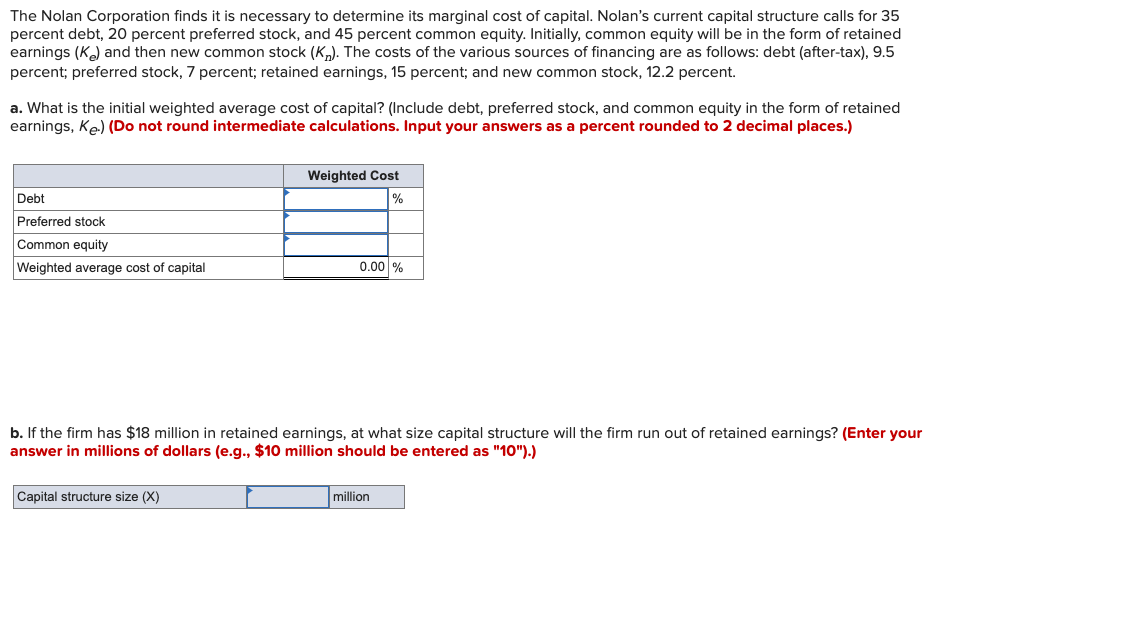The Nolan Corporation finds it is necessary to determine its marginal cost of capital. Nolan's current capital structure calls for 35 percent debt, 20 percent preferred stock, and 45 percent common equity. Initially, common equity will be in the form of retained earnings (Ke) and then new common stock (Kn). The costs of the various sources of financing are as follows: debt (after-tax), 9.5 percent; preferred stock, 7 percent; retained earnings, 15 percent; and new common stock, 12.2 percent. a....

• ### Problem 11-28 Marginal cost of capital (LO11-5) 1.7 The Nolan Corporation finds it is necessary to...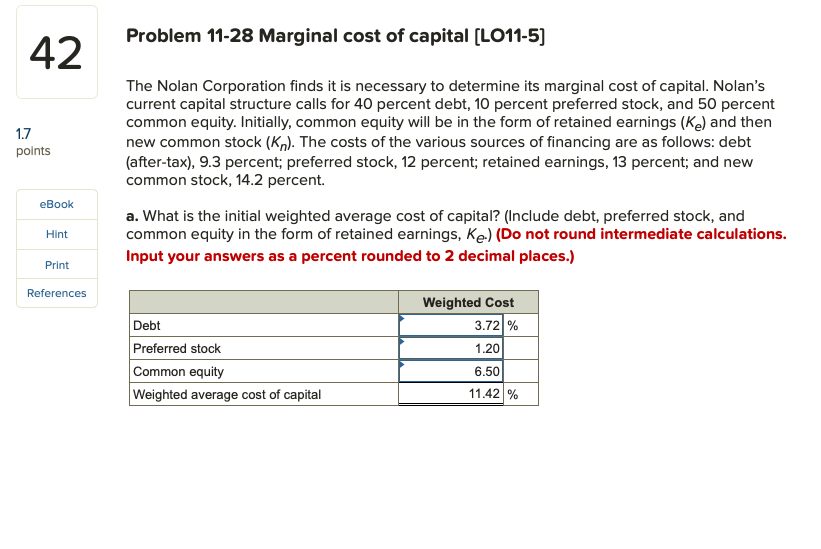Problem 11-28 Marginal cost of capital (LO11-5) 1.7 The Nolan Corporation finds it is necessary to determine its marginal cost of capital. Nolan's current capital structure calls for 40 percent debt, 10 percent preferred stock, and 50 percent common equity. Initially, common equity will be in the form of retained earnings (Ke) and then new common stock (Kn). The costs of the various sources of financing are as follows: debt (after-tax), 9.3 percent; preferred stock, 12 percent; retained earnings, 13...

• ### The McGee Corporation finds it is necessary to determine its marginal cost of capital. McGee's current...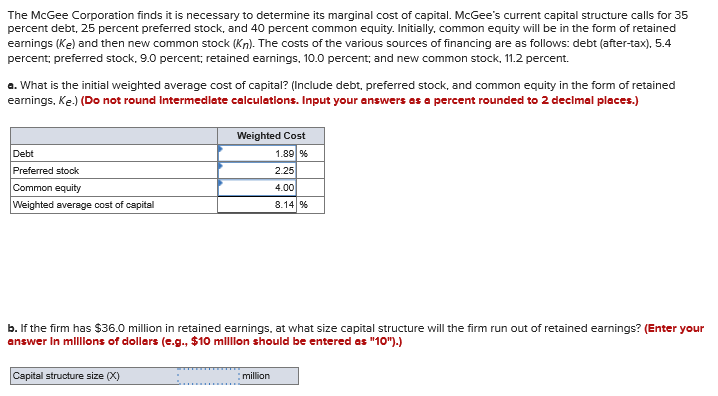The McGee Corporation finds it is necessary to determine its marginal cost of capital. McGee's current capital structure calls for 35 percent debt, 25 percent preferred stock, and 40 percent common equity. Initially, common equity will be in the form of retained earnings (Ke) and then new common stock (Kn). The costs of the various sources of financing are as follows: debt (after-tax), 5.4 percent, preferred stock, 9.0 percent; retained earnings, 10.0 percent; and new common stock, 11.2 percent. a....

• ### The McGee Corporation finds it is necessary to determine its marginal cost of capital. McGee's current capital structu...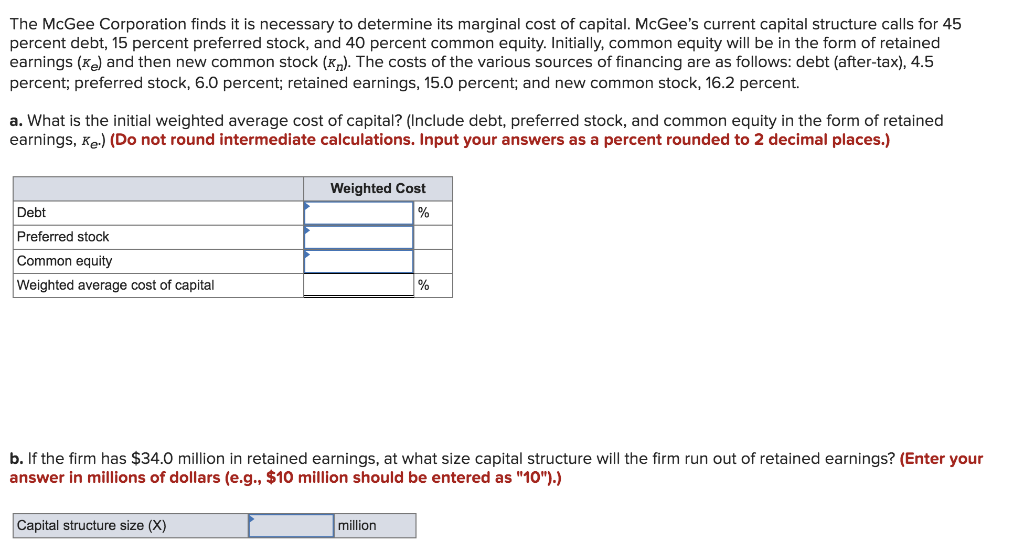The McGee Corporation finds it is necessary to determine its marginal cost of capital. McGee's current capital structure calls for 45 percent debt, 15 percent preferred stock, and 40 percent common equity. Initially, common equity will be in the form of retained earnings (K and then new common stock (K,). The costs of the various sources of financing are as follows: debt (after-tax), 4.5 percent; preferred stock, 6.0 percent; retained earnings, 15.0 percent; and new common stock, 16.2 percent a....

• ### The McGee Corporation finds it is necessary to determine its marginal cost of capital. McGee’s current capital structure calls for 40 percent debt, 5 percent preferred stock, and 55 percent common equity. Initially, common equity will be in the form of re

What is the initial weighted average cost of capital? (Include debt, preferred stock, and common equity in the form of retained earnings, Ke.) (Do not round intermediate calculations. Input your answers as a percent rounded to 2 decimal places.)  b. If the firm has \$22.0 million in retained earnings, at what size capital structure will the firm run out of retained earnings? (Enter your answer in millions of dollars (e.g., \$10 million should be entered as "10").)  c. What will the marginal cost of capital be immediately...